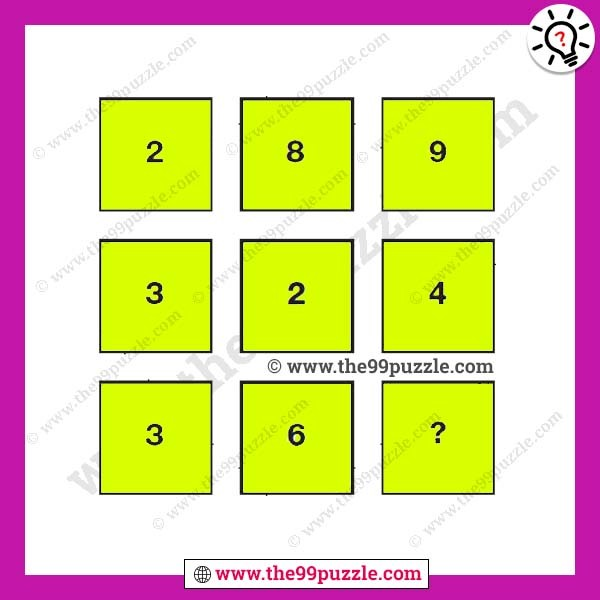This missing number puzzle for adults. Can you solve this number puzzle? In this reasoning math puzzle picture, you get numbers and boxes in mathematical logic. Can you find the math logic and replace the question mark. When you try to solve this type of puzzle, think logically and mathematically. This type of puzzle is asked in many interviews and examinations. Now, solve this missing number puzzle and replace the question mark quickly.###### Explanation:

Reading each row as a 3 digit number, the rows follow the sequence of square numbers, from 17 to 19.